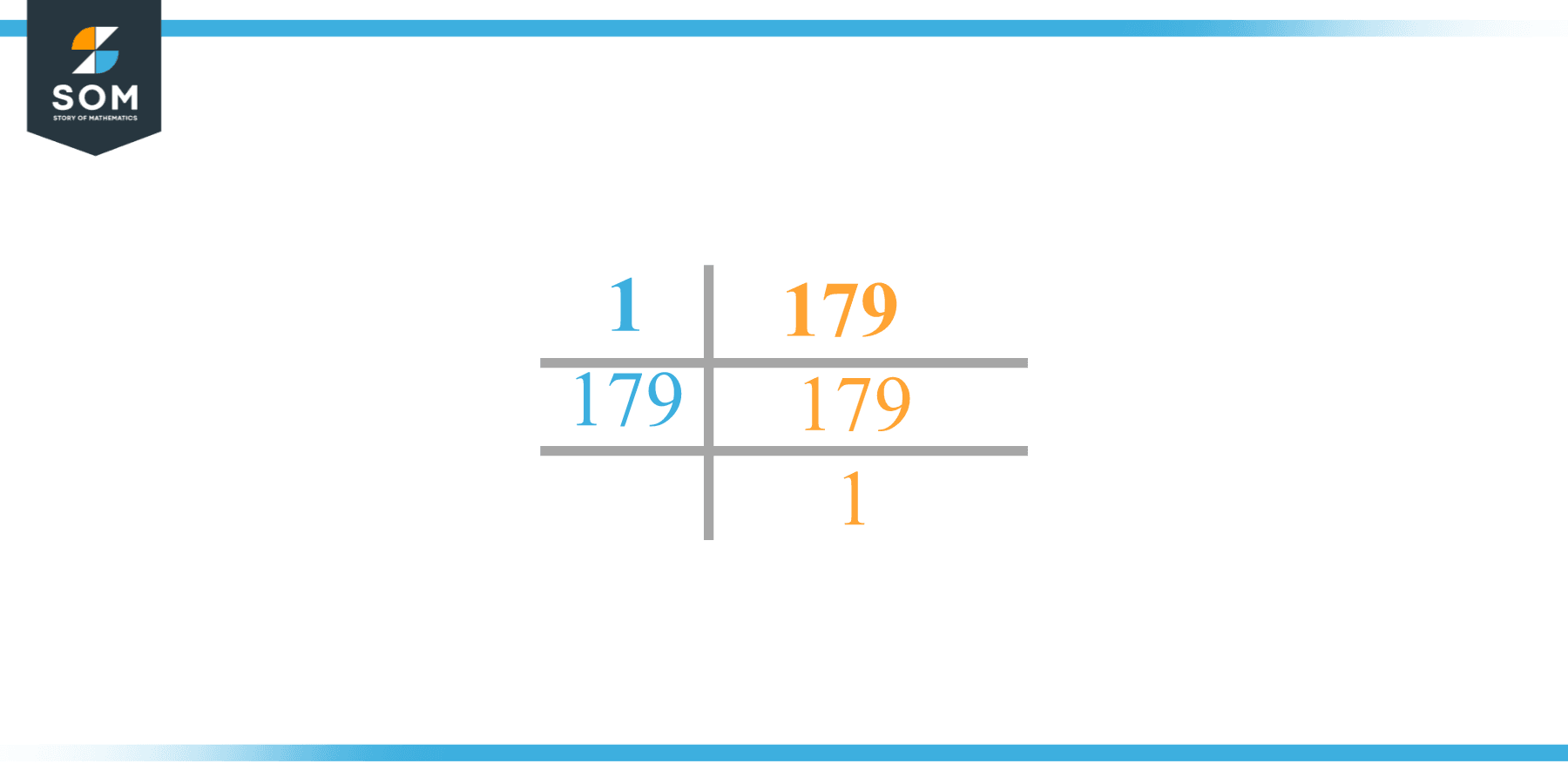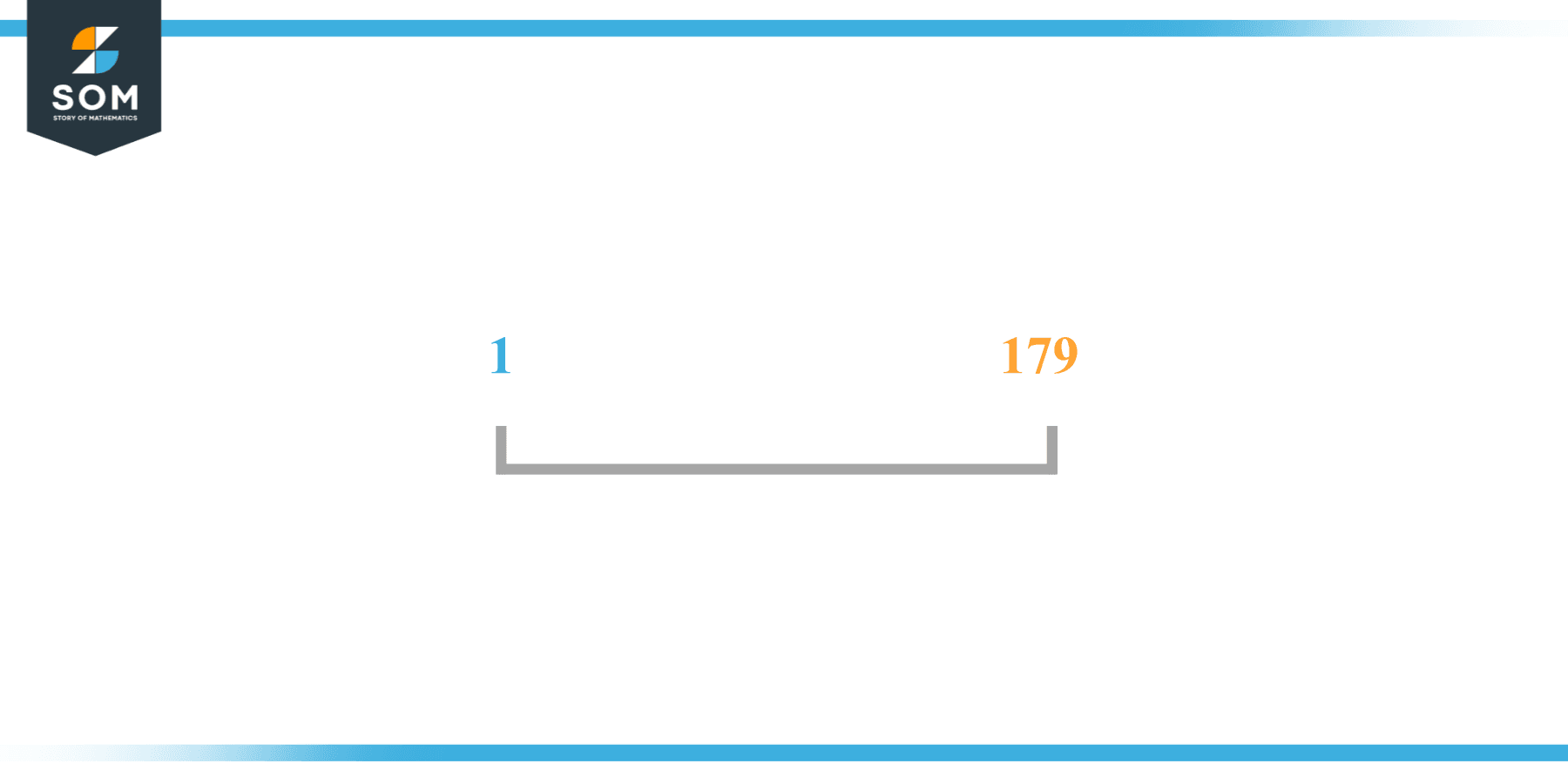# Factors of 179: Prime Factorization, Methods, and Examples

The factors of 179 are the whole numbers that when divided by 179 leave nothing as the residue. 179 is an odd number. It is also a prime number due to which it has only two factors. This means the numbers that completely divide the given number are named as its factors.The factors of the given number can be positive as well as negative provided that the given number is achieved upon multiplication of two-factor integers.

### Factors of 179

Here are the factors of number 179.

Factors of 179: 1, 179

### Negative Factors of 179

The negative factors of 179 are similar to its positive factors, just with a negative sign.

Negative Factors of 179: -1, -179

### Prime Factorization of 179

The prime factorization of 179 is the way of expressing its prime factors in the product form.

Prime Factorization: 1 x 179

In this article, we will learn about the factors of 179 and how to find them using various techniques such as upside-down division, prime factorization, and factor tree.

## What Are the Factors of 179?

The factors of 179 are 1 and 179. All of these numbers are the factors as they do not leave any remainder when divided by 179.

The prime factors of the number 179 can be determined using the technique of prime factorization.

## How To Find the Factors of 179?

You can find the factors of 179 by using the rules of divisibility. The divisibility rule states that any number, when divided by any other natural number, is said to be divisible by the number if the quotient is the whole number and the resulting remainder is zero.

To find the factors of 179, create a list containing the numbers that are exactly divisible by 179 with zero remainders. One important thing to note is that 1 and 179 are the 179’s factors as every natural number has 1 and the number itself as its factor.

1 is also called the universal factor of every number. The factors of 179 are determined as follows:

$\dfrac{179}{1} = 179$

Therefore, 179 has only two factors that are 1 and 179.

### Total Number of Factors of 179

For 179 there are 2 positive factors and 2 negative ones. So in total, there are 4 factors of 179.

To find the total number of factors of the given number, follow the procedure mentioned below:

1. Find the factorization of the given number.
2. Demonstrate the prime factorization of the number in the form of exponent form.
3. Add 1 to each of the exponents of the prime factor.
4. Now, multiply the resulting exponents together. This obtained product is equivalent to the total number of factors of the given number.

By following this procedure the total number of factors of 179 is given as:

Factorization of 179 is 1 x 179.

The exponent of  1 and 179 is 1.

Adding 1 to each and multiplying them together results in 4.

Therefore, the total number of factors of 179 is 4. 2 are positive and 2 factors are negative.

### Important Notes

Here are some important points that must be considered while finding the factors of any given number:

• The factor of any given number must be a whole number.
• The factors of the number cannot be in the form of decimals or fractions.
• Factors can be positive as well as negative.
• Negative factors are the additive inverse of the positive factors of a given number.
• The factor of a number cannot be greater than that number.
• Every even number has 2 as its prime factor, the smallest prime factor.

## Factors of 179 by Prime FactorizationThe number 179 is a prime number. Prime factorization is a useful technique for finding the number’s prime factors and expressing the number as the product of its prime factors.

Before finding the factors of 179 using prime factorization, let us find out what prime factors are. Prime factors are the factors of any given number that are only divisible by 1 and themselves.

To start the prime factorization of 179, start dividing by its smallest prime factor. First, determine that the given number is either even or odd. If it is an even number, then 2 will be the smallest prime factor.

Continue splitting the quotient obtained until 1 is received as the quotient. The prime factorization of 179 can be expressed as:

179 = 1 x 179

## Factors of 179 in PairsThe factor pairs are the duplet of numbers that when multiplied together result in the factorized number. Factor pairs can be more than one depending on the total number of factors of the given numbers.

As 179 is a prime number it has only two factors in total therefore it can make only 1 pair of factors.

1 x 179 = 179

The possible factor pair of 179 is given as (1, 179).

The negative factor pair of 179 is given as:

-1 x -179 = 179

It is important to note that in negative factor pairs, the minus sign has been multiplied by the minus sign due to which the resulting product is the original positive number. Therefore, -1 and -179 are called negative factors of 179.

The list of all the factors of 179 including positive as well as negative numbers is given below.

Factor list of 179: 1, -1, 179, and -179

## Factors of 179 Solved Examples

To better understand the concept of factors, let’s solve some examples.

### Example 1

How many factors of 179 are there?

### Solution

The total number of Factors of 179 is 2.

Factors of 179 are 1 and 179

### Example 2

Find the factors of 179 using prime factorization.

### Solution

The prime factorization of 179 is given as:

179 $\div$ 1 = 179

So the prime factorization of 179 can be written as:

1 x 179 = 179